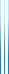# Courses

236646 Boolean Function Analysis Spring 2021

Boolean function analysis is the study of functions on the Boolean cube $\{\pm1\}^n$ and related domains from a spectral perspective. It has become an essential tool in many areas of theoretical computer science, combinatorics, and probability theory.

Lectures will be given online on Zoom. The Zoom link is available on webcourse.

I have prepared lecture notes. Bonus: Exposition of the Keller–Klein proof of the Kinlder–Safra theorem.

Topics covered in the lecture notes include:

• Classical stuff:
• Basic definitions: Fourier expansion, influence, noise.
• Basic results: Friedgut–Kalai–Naor theorem, Kindler–Safra theorem, Kahn–Kalai–Linial theorem, Friedgut’s junta theorem.
• Hypercontractivity.
• Invariance principle, Majority is Stablest, Bourgain’s junta theorem.
• $p$-biased analysis and the Russo–Margulis formula.
• Applications:
• Linearity testing.
• Approximate polymorphisms.
• Consistent labelling, a type of list-decoding crucial for applications in hardness of approximation.
• $p$-biased analysis for small $p$: Friedgut–Kalai–Naor theorem, global hypercontractivity, Bourgain’s sharp threshold theorem.
• Analysis on the slice.

Topics covered in class:

1. March 21: Introduction and linearity testing (Section 1).
2. April 4: Polymorphisms of Majority (Section 2 up to Section 2.1). Bonus: open question on Boolean degree 1 functions on the Grassmann scheme.
3. April 11: Approximate polymorphisms of Majority (Section 2.1).
4. April 18: Friedgut–Kalai–Naor theorem (Section 3). Bonus: Kindler–Safra theorem and Bourgain’s junta theorem.
5. April 25: Influence (Section 4).
6. May 2: Friedgut’s theorem and the KKL theorem (Section 5).
7. May 9: Hypercontractivity (Section 6 up to Section 6.1; time permitting, Section 6.1).
8. May 23: Biased Fourier expansion and Erdős–Ko–Rado (Section 8, Section 8.1).
9. May 30: Biased hypercontractivity (Section 8.2), Russo–Margulis lemma, sharp threshold theorems (Section 9 up to Section 9.1).
10. June 6: Biased FKN theorem (Section 10).
11. June 13: Invariance principle (Section 11, Section 11.1).
12. June 20: Global hypercontractivity (Section 12, Section 12.1).
13. June 27: Analysis on the slice (parts of Section 13).

Assignments:

## OTHER SEMESTERS

• 236648 Boolean Function Analysis Spring 2019
• 236646 Boolean Function Analysis Winter 2015/2016